Bart M. ter Haar Romeny

## Dither RemovalNBCDFPDF

Mathematica is ideal for explaining the design of seemingly complex mathematical methods. In this article we explain the use of the 2D Fourier transform to remove unwanted dithering artifacts from images. All steps are visualized, so the reader can get a good idea of what the Fourier transform of an image looks like, the location of the origin, the artifacts and their extent, and how geometric reasoning works in the Fourier domain. The method leads to a marked clean up of images deteriorated by dither.

### Image Dithering

Image dithering is often used for commercial print products like newspapers and magazines. Many printers (e.g., laser and inkjet printers) can only put a series of single black dots on paper. Intensity is then regulated by the local density of the dots. This process is called dithering. However, when a dithered image is scanned, the interaction between the discrete scanning process and dithering may seriously degrade the image. Dithering and scanning are often both periodic, leading to periodic interference artifacts. These can be removed by proper filtering in the spatial 2D Fourier domain.

We first give an example of how dithering can be applied to an image. It is simplest to replace each pixel with a small array of random black dots, with the number of dots proportional to the intensity.

Some general settings: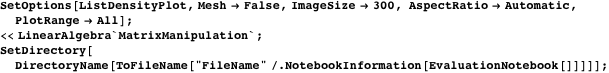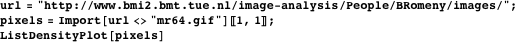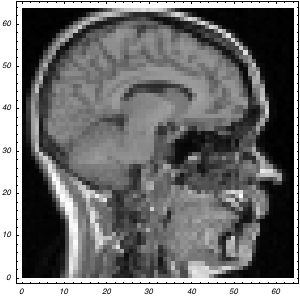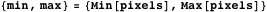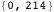The dithering function creates a small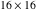array, which enables us to make 256 different intensities.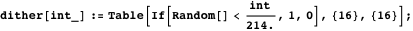We map this onto every pixel and get an image that is 16 times larger with dithered points. The function BlockMatrix (available in LinearAlgebra`MatrixManipulation`) combines the different submatrices.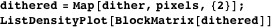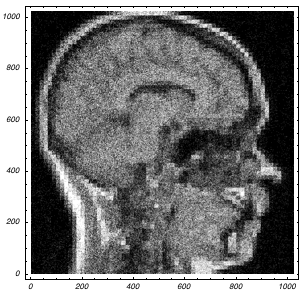There are many sophisticated ways to dither an image. For a good overview see . One of the best methods is the Floyd-Steinberg dithering method, based on error-diffusion . The following graphic is an example.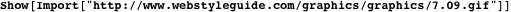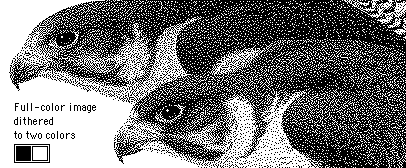### Scanning a Dithered Image

A problem occurs when the dithering mask is not made up of randomly placed black-and-white pixels but has some regularity. The dithered image then contains many highly regular spatial frequencies, which may give rise to interference patterns when the image is sampled with a regular scanner, which likely has a different sampling frequency. The result is a range of extra harmonics (i.e., frequencies that are the result of the interference). They come as the products of the frequencies involved. An example of the period of the frequencies that emerge follows.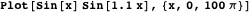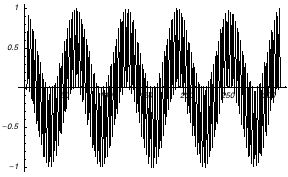This occurs because the product signal is actually the addition of the sum and the difference of the base frequencies.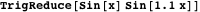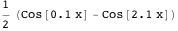The lower frequencies are rarely a problem. The higher frequencies can be removed by proper low-pass filtering.

### Dither Removal by Filtering

A “large” dithered and scanned input image follows (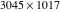pixels, 1.9MB). Note the periodic artifacts.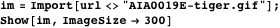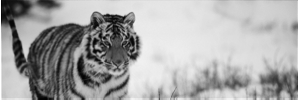Figure 1. Image of a tiger, produced by printing a stock-art photo with an 85 lines per inch dot screen printer and then scanning.

The image is imported as a structure, in which the pixels and the header data are embedded. The pixels can be extracted as the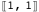element.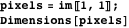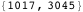To develop the method, we do not need the whole image. For speed and lower memory use, we take a subimage. We can see the periodic dithering more clearly by zooming in.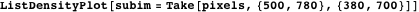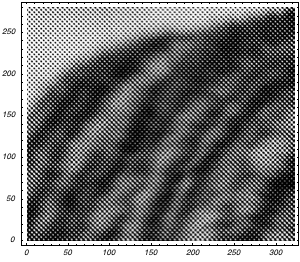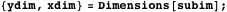Because the dithering has a high spatial frequency, we suspect these frequencies to be higher than most of the other frequencies in the image. We compute the Fourier spectrum of the input image and plot its absolute value. Because the origin is really large, the image is automatically scaled with a large factor, which reduces the contrast. We zoom in on a limited range (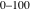) of spectral values.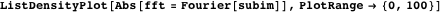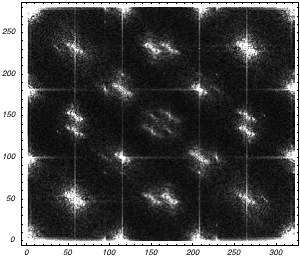The significant image frequencies are around the origin (i.e., in the corners of the periodic spectrum plot). The marked peaks at other locations are proof of the existence of strong periodic signals due to dithering. We will use a mask that allows only frequencies near the origin to be included: a low-pass filter for spatial frequencies.

The mask is most easily created by an elliptic Gaussian weighting function in the center (different standard deviations in theanddirections), which is then shifted over half the image size in each dimension to put the kernel at the origin of the Fast Fourier Transform (FFT) domain, which is in the lower left. Note that the Fourier domain is periodic in either dimension, so we actually see a single tile of a whole tiled 2D space in theanddirections. The standard deviations have to be estimated from the 2D plot of the spectrum. For speed, we exploit the separability of the 2D Gaussian kernel. We first make two 1D arrays of Gaussian values and then multiply them into a matrix with Outer in a compiled function. This is about 140 times faster than a 2D Table command.xs andys are the standard deviations of the Gaussian in theanddirections of the subimage.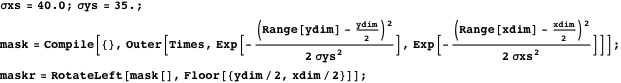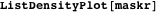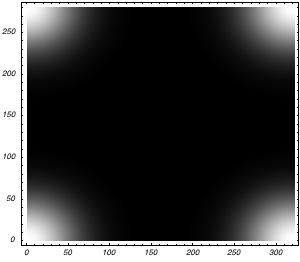We also can inspect the amplitude spectrum and the low-pass Gaussian filter (both functions are scaled for plotting) along the interpolated diagonal positions of the rectangular image.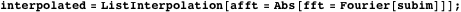Here we view the mask as a height plot.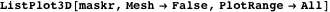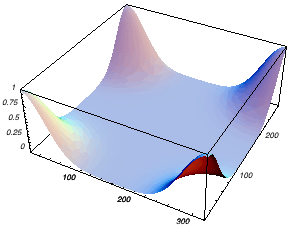We multiply the Fourier spectrum with the mask and study it visually.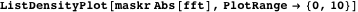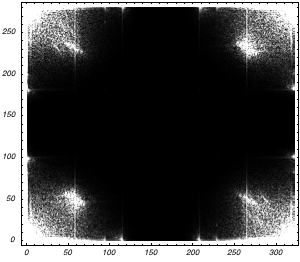We mask out the unwanted frequencies by multiplying the spectrum with the mask, and we get the resulting image by the inverse Fourier transform. The Gaussian filtering is still letting some periodic frequencies through (we only like to keep the information near the corners), so we make it steeper by raising it to the third power.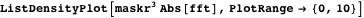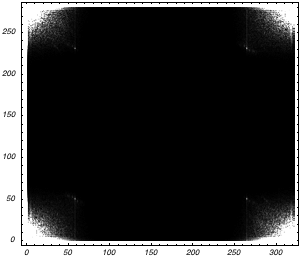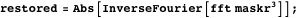The result is a markedly improved image, without the disturbing dithering frequencies.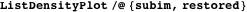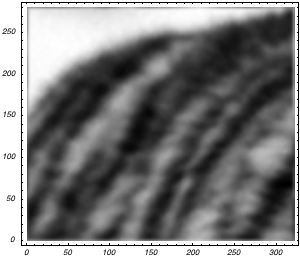The dither is clearly removed, and details, such as the local hair bundles, can now clearly be discriminated.

The following reads the whole image, processes the data in the Fourier domain (note that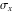and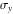are proportionally rescaled), and writes the restored image to disk, in this case as a GIF file.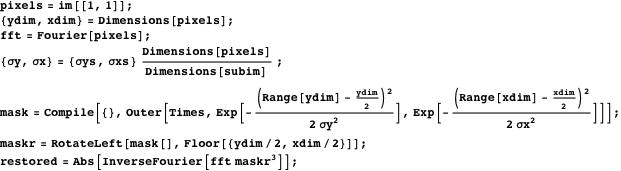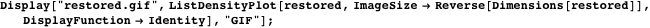### Conclusion

This example shows the use of Fast Fourier Transform to remove periodic artifacts from an image. Despite the large size of the input image, the numerical performance of Mathematica is good.

### References

  R. L. Adler, B. P. Kitchens, M. Martens, C. P. Tresser, and C. W. Wu, “The Mathematics of Halftoning,” IBM Journal of Research and Development, 47(1), 2003 pp. 5-15 www.research.ibm.com/journal/rd/471/adler.pdf.  R. Floyd and L. Steinberg, “An Adaptive Algorithm for Spatial Grey Scale,” SID International Symposium Digest of Technical Papers, San Jose, CA: The Society for Information Display (SID), 1975 pp. 36-37. B. M. ter Harr Romeny, “Dither Removal,” The Mathematica Journal, 2012. dx.doi.org/10.3888/tmj.10.2-9.